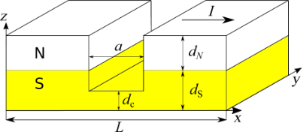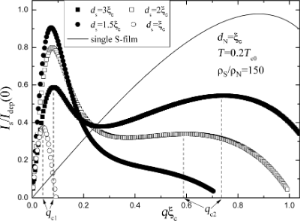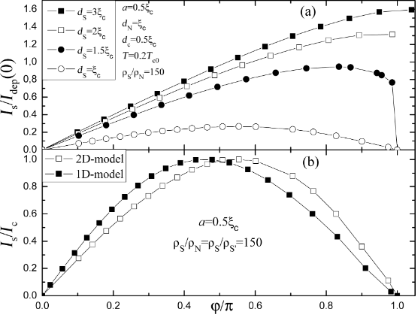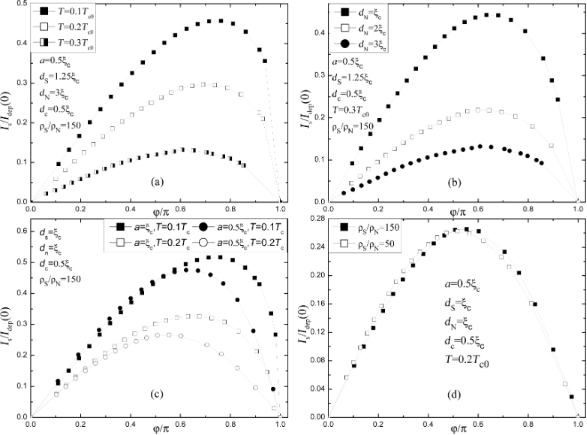# A Josephson junction based on a highly disordered superconductor/low-resistivity normal metal bilayer

Institute for Physics of Microstructures, Russian Academy of Sciences, Nizhny Novgorod, 603950, Russia

1. Corresponding author email

Guest Editor: A. S. Sidorenko
Beilstein J. Nanotechnol. 2020, 11, 858–865. https://doi.org/10.3762/bjnano.11.71
Received 20 Mar 2020, Accepted 22 May 2020, Published 02 Jun 2020

A non-peer-reviewed version of this article has been posted as a preprint https://arxiv.org/abs/2002.07552

• Full Research Paper

## Abstract

We calculate the current–phase relation (CPR) of a SN-S-SN Josephson junction based on a SN bilayer of variable thickness composed of a highly disordered superconductor (S) and a low-resistivity normal metal (N) with proximity-induced superconductivity. In such a junction, the N layer provides both a large concentration of phase in the weak link and good heat dissipation. We find that when the thickness of the S and the N layer and the length of the S constriction are about the superconducting coherence length the CPR is single-valued and can be close to a sinusoidal shape. The product IcRn can reach Δ(0)/2|e| (Ic is the critical current of the junction, Rn is its normal-state resistance, Δ(0) is the superconductor gap of a single S layer at zero temperature). Our calculations show, that the proper choice of the thickness of the N layer leads both to nonhysteretic current–voltage characteristics even at low temperatures and a relatively large product IcRn.

Keywords: normal metal–superconductor bilayer; Josephson junction; Joule heating

## Introduction

Josephson junctions are of interest for applications such as voltage standards , SQUID magnetometers , particle detectors , and energy-efficient superconductor logic and memory circuits [4,5]. These applications need to have a large critical current Ic to achieve high noise immunity. Also many of these applications require to have a nonhysteretic current–voltage characteristic (IVC) and a large characteristic voltage Vc = IcRn, where Rn is the normal-state resistance of the junction.

Tunnel superconductor–insulator–superconductor (SIS) Josephson junctions are characterized by small critical current densities (significantly smaller than the depairing current density of superconducting electrodes) and a hysteretic IVC (the latter is related with the large capacitance of the insulator layer), which restricts their applicability. Elimination of hysteresis in SIS junctions requires an external resistor or a more complex circuitry. S-c-S Josephson junctions (where “c” is a geometric constriction) have a small capacitance of the weak link and a high critical current (about the magnitude of the depairing current of a superconductor), which allows one to obtain high noise immunity. But due to large critical current and bad heat dissipation their IVCs are hysteretic due to Joule heating () and the subsequent formation of a stable region with suppressed superconductivity (a so-called “hot spot”) at I > Ic [6-9]. At temperatures near the critical temperature Tc the hysteresis is absent because of the low Ic and, therefore, small dissipation, but this leads to a small voltage Vc.

Therefore, eliminating the thermal hysteresis without sacrificing the voltage Vc is important, albeit a nontrivial problem. One solution is a normal metal shunt either on top of the junction  or at a distance from it [11,12]. The resistance and the position of the shunt play an important role and they can lead to a reduction of the junction characteristics because of the proximity effect or a very small shunt resistance. In [13,14], it was proposed to use a variable-thickness SN-N-SN bilayer in which the superconducting layer is partially (or entirely) etched by means of a focused ion beam. A sufficiently thick normal metal layer act as a good thermal bath, which yields a nonhysteretic current–voltage characteristic even at low temperatures. However, the increase of the thickness of the N layer leads to a significant decrease of Rn and, hence, to smaller values of Vc.

In our work, we calculate the current–phase relation and heating effects in SN-S-SN Josephson junctions of variable thickness based on a thin dirty superconductor with large normal-state resistivity, ρS ≥ 100 μΩ·cm, and a thin normal metal layer with low resistivity, ρN ≥ 2 μΩ·cm. In such a thin SN bilayer the superconducting current mainly flows in the N layer (due to proximity-induced superconductivity and ρSN ≫ 1), and the critical current of the SN bilayer may exceed the critical current of a single S layer if the thickness of the S and the N layers are about the superconducting coherence length . Because of the large diffusion coefficient, DNDS, the N layer provides both a large phase concentration in the constriction leading to a single-valued current–phase relation (CPR) and an effective thermal bath into which the heat from the junction area could be dissipated, resulting in nonhysteretic IVC even at relatively low temperatures. We also find that in comparison with a SN-N-SN junction, the critical current density could be similar to the depairing current density of the S layer, which makes it possible to obtain IcRn ≈ Δ(0)/2|e|.

## Model

The model system consists of a SN bilayer strip with length L made of a superconducting film with thickness dS and a normal metal film with thickness dN. At the center of the bilayer there is a constriction with length a and thickness dc where the N layer and, partially, the S layer are removed (Figure 1).Figure 1: Sketch of a SN-S-SN Josephson junction based on a SN strip of variable thickness. Figure 1: Sketch of a SN-S-SN Josephson junction based on a SN strip of variable thickness. Jump to Figure 1

We assume that in our system the current flows in the x direction, and in the y direction the system is uniform. To find the current–phase relation of such a SN-S-SN Josephson junction at all temperatures below Tc we solve a 2D Usadel equation for quasiclassical normal g and anomalous f Green’s functions. With the angle parametrization g = cos Θ and f = sin Θ exp(iϕ), the 2D Usadel equation in different layers can be written as

where the subscripts S and N refer to the superconducting and the normal layer, respectively. Here ℏωn = πkBT(2n + 1) are the Matsubara frequencies (n is an integer number), q = ∇ϕ = (qx, qz) is the quantity that is proportional to the supervelocity vs, and ϕ is the phase of the superconducting order parameter. Δ is the magnitude of the order parameter, which should satisfy the self-consistency equation

where Tc0 is the critical temperature of the single S layer. We assume that Δ is nonzero only in the S layer because of the absence of attractive phonon-mediated electron–electron coupling in the N layer. Equation 1 and Equation 2 are supplemented by the Kupriyanov–Lukichev boundary conditions  between the layers:

In the model we assume a transparent interface between the N and the S layer, which leads to the continuity of Θ at the NS boundary. At the boundaries of the system with the vacuum we use dΘ/dn = 0.

To find the phase distribution ϕ Equation 1Equation 3 are supplemented by a 2D equation,

Here, js is the superconducting current density, which is determined by the following expression:

where ρ is the residual resistivity of the corresponding layer. At the SN-interface we use a boundary condition similar to Equation 4, and for the interfaces with the vacuum we use dϕ/dn = 0. At the system ends rigid boundary conditions are imposed:

where δϕ is the fixed phase difference between the system ends. This is different from the phase drop near the junction, which we define as

where k = qx (x = 0) is far from the constriction (in a similar way φ is defined in [17,18]). The value of k is found from the self-consistings solution of Equation 1Equation 3 and Equation 5.

In numerical calculations we use dimensionless units. The magnitude of the order parameter is normalized by kBTc0 = Δ(0)/1.76, lengths are in units of≈ 1.33ξ(0), whereis the superconducting coherence length at T = 0, and the current is in units of the depairing current Idep of the superconductor at T = 0.

To calculate the CPR we numerically solve Equation 1Equation 3 and Equation 5 by using an iteration procedure with fixed δϕ. When self-consistency is achieved (we stop the calculations when the maximal relative change of Δ between consequent iterations is less than 10−4) the Green’s functions are used to calculate js and the supercurrent per unit of width, Is:

We also compare the calculated CPR with the CPR of a 1D S’-S-S’ system with a large ratio between the diffusion coefficients DS′/DS ≫ 1 (the length of the superconductor S is equal to a). For these calculations we use a 1D Usadel equation.

## Results

### Current–phase relation of the SN-S-SN Josephson junction

The function Is(q) in the SN bilayer may have one or two maxima depending on the value of dS (see Figure 2) or of dN (see Figure 3a in ). The maximum at small q is connected with the suppression of proximity-induced superconductivity in the N layer atwhile the second maximum atcomes from the suppression of superconductivity in the S layer when q > qc2. The large difference between qc1 and qc2 leads to a larger phase concentration in the S constriction (see Figure 1) in comparison with the variable-thickness strip (or Dayem bridge) made of the same material and having the similar geometrical parameters. Because of that, for relatively thin S layers the CPR is single-valued (see Figure 3a), which is not easy to achieve in a Dayem bridge . For relatively large dS there is a noticeable contribution to the total supercurrent from the S layer, which means a smaller current (phase) concentration in the constriction like in a common Dayem bridge, and the CPR becomes multi-valued (see Figure 3a for dS = 2ξc and 3ξc).Figure 2: Dependence of the superconducting current Is flowing along the SN bilayer on q for different dS. The solid line shows the dependence of Is on q for the single S strip. The dashed lines show the critical values of q. The current is normalized by the depairing current Idep of the single S strip with thickness dS at T = 0. Figure 2: Dependence of the superconducting current Is flowing along the SN bilayer on q for different dS. Th... Jump to Figure 2Figure 3: (a) Current–phase relation of a SN-S-SN Josephson junction at different dS. The current is normalized by the depairing current Idep of the single S strip with thickness dc at T = 0. The junction parameters are shown in the figure. (b) Comparison of current–phase relations calculated on the basis of 1D and 2D models. For the 2D case the parameters are dS = dN = ξc, dc = 0.5ξc, T = 0.2Tc0. For the 1D case the temperature T = 0.6Tc0 corresponds to T = 0.6Tc, where Tc = 0.32Tc0 is the critical temperature of the SN bilayer with the chosen parameters. The superconducting current is normalized by the critical current of the Josephson junction. Figure 3: (a) Current–phase relation of a SN-S-SN Josephson junction at different dS. The current is normaliz... Jump to Figure 3

In some respect, the studied Josephson junction resembles Josephson junctions based on a S’-S-S’system composed of two superconductors S and S’ having DS′DS and the same thicknesses dS = dS′ [17,20,21]. A Josephson junction based on this quasi 1D system has a single-valued CPR, which approaches a sinusoidal shape with increasing temperature. In Figure 3b we compare the CPRs calculated for 1D S’-S-S’ and 2D SN-S-SN systems. Since in the 1D model there is no suppression of Tc through the N layer, we use in the calculations the ratio T/Tc0, which corresponds to the ratio T/Tc of the 2D SN structure. Visible differences between the calculated CPRs using different models could be related with a transversal inhomogeneity near the S constriction in the 2D case.

We have also studied the evolution of the CPR of the SN-S-SN Josephson junction when varying different parameters. In Figure 4a we demonstrate that with increase of the temperature the current–phase relation comes close to a sinusoidal shape. At T = 0.3Tc0 the amplitude of the first harmonic, sin ϕ, is 0.98Ic and the amplitude of the second harmonic, sin 2ϕ, is −0.19Ic). This is typical for S’-S-S’ junctions  and is related to the increase of the temperature-dependent coherence length ξ(T). The effect of different dN is shown in Figure 4b. An increase in dN leads to a slight shift of the maximum of Is(φ) to the left and a decrease of Ic. This can be explained by a lowering of Tc of the SN bilayer for thicker N layers. A lower Ic means smaller values of IcRn. However, as we discuss below, a large value of dN provides better cooling of the S constriction and nonhysteretic IVCs.Figure 4: Variation of current–phase relation of SN-S-SN junction as a function of: (a) the temperature; (b) the thickness of the N layer dN; (c) the length of the constriction, a; (d) the ratio between the resistivities. The current is normalized by the depairing current Idep of the superconducting strip with thickness dc at T = 0. Figure 4: Variation of current–phase relation of SN-S-SN junction as a function of: (a) the temperature; (b) ... Jump to Figure 4

An increase of the length of the weak link, a, leads to the shift of the maximum of Is(φ) to the right (see Figure 4c) as it is typical for common Josephson junctions with variable thickness. Interestingly, in contrast to common junctions, Ic increases in the SN-S-SN system. This can be explained by a lower value of the superconducting order parameter in SN banks in comparison with Δ in the S constriction at Is = 0. With increasing a the superconducting order parameter in the constriction increases and Ic increases too.

Finally, Figure 4d illustrates that a decrease of the ratio ρSN to a third of the initial value hardly changes the current–phase relation. Both the critical current and the shape of the CPR vary only little.

### Effect of Joule heating in SN-S-SN junctions

The absence of hysteresis in the current–voltage characteristics is important for devices based on Josephson junctions. The hysteresis in Dayem bridge, variable-thickness, S’-S-S’ or S-N-S junctions is mainly caused by the temperature rise in the weak-link region in the resistive state due to Joule heating and the formation of hot spots [7-9]. A relatively large gap Δ in superconducting banks plays an important role here because it prohibits heat dissipation from the S or the N link at low temperatures kBT < Δ and it leads to hysteresis even for S-N-S junctions of variable thickness . This problem could be resolved by adding heat sinks (voltage leads attached to the N link could play such a role ). However, this complicates the geometry of the junction. Local heat production is expected to be large in a SN-S-SN junction due to large critical current density, which is about the depairing current density of the superconductor. As we show below, the presence of a relatively thick N layer with large diffusion coefficient and small minigap in the electron spectra provides efficient cooling of the constriction.

To estimate the increase of temperature in the resistive state we use a two-temperature (2T) model [24,25] for the SN-S-SN junction. We suppose that electron temperature Te = T + δTe and phonon temperature Tp = T + δTp are close to the substrate temperature, δTe, δTpT and do not vary along the thickness. In the N layer the proximity-induced gap (minigap) is small, and, due to the inverse proximity effect, the gap in the relatively thin S layer (dS ≤ 1.5ξc) is also suppressed in comparison with a single S layer, which permits heat diffusion from the N to the S layer in SN banks. In the S constriction being in the resistive state at I > Ic the superconducting order parameter is also suppressed. It allows us to use normal-state heat conductivity both in the SN and the S region in the heat conductance equation for the calculation of δTe. This is in contrast to S-N-S and S’-S-S’ junctions where heat conductivity is suppressed in the superconducting banks. In our model Joule dissipation is taken into account only in the S constriction, because in the SN bilayer it is considerably lower due to the much lower resistivity and lower current density. Because of the small length of the constriction and the large difference in diffusion coefficients and thicknesses in constriction and banks we can neglect heat flow to phonons and substrate in the constriction (the main cooling of the junction comes from the diffusion of hot electrons to SN banks). In the SN bilayer DNDS and heat diffusion occurs mainly along the N layer. With above assumptions we obtain the following equation for δTe:

whereis the electron heat conductivity of the S layer in the normal state, and N(0) is the one-spin density of states on the Fermi level,

is the thermal healing length, β = [γτesc 450ζ(5)T/[τ0π4Tc0], ζ(5) ≈ 1.03, τesc is the escape time of nonequilibrium phonons to the substrate, γ = 8π2Ce(Tc0)/Cp(Tc0) is the ratio between electron and phonon heat capacity at T = Tc0 and τ0 determines the strength of electron–phonon inelastic scattering in the S and the N layer (see Equations 4 and 6 in ). For τ0 we use the smallest time for S and N materials due to the assumably good transfer of electrons between the S and the N layer and the small thickness of the layers. On the boundary between S and SN regions we use a continuity of the electron temperature, δTe|a/2−0 = δTe|a/2+0, and of the heat fluxUsing Equation 10 and above boundary conditions, we find the maximal temperature increase in the constriction:

In the following estimations we use the parameters of NbN (S layer) and Cu (N layer): Tc0 = 10 K, DS = 0.5 cm2/s, ρS = 200 μΩ·cm, DN = 40 cm2/s, ρN = 2 μΩ·cm, τ0 = 1 ns (theoretical estimation for NbN taken from ), ξc = 6.4 nm, γ = 9, dS = 1.25ξc, dN = 2ξc, τesc = 4(dN + dS)/u ≈ 41 ps (u = 2·105 cm2/s is the mean speed of sound), T/Tc0 = 0.3, Tc/Tc0 = 0.43, a= 0.5ξc, dc = 0.5ξc. With these parameters β ≈ 0.53, Ic ≈ 0.22Idep(0) (see Figure 4b) andis small, thanks to DNDS and dNdc.

## Discussion

We use an Usadel model to calculate the current–phase relation of a SN-S-SN Josephson junction based on a high-resistivity superconductor and a low-resistivity normal metal. In , from comparison of experiment and theory it was concluded that the Usadel model underestimates proximity-induced superconductivity in the N layer and overestimates the inverse proximity effect in the S layer in NbN/Al, NbN/Ag and MoN/Ag bilayers. Namely, the suppression of the critical temperature of the SN bilayer is smaller while the change in magnetic field penetration depth of the SN bilayer is larger than the Usadel model predicts. Therefore, the present results should be considered only as a route for a possible experimental realization of SN-S-SN Josephson junctions. They demonstrate that the thickness of the S layer should not exceed ca. 1.5ξc, otherwise the current–phase relation is not single-valued for reasonable lengths and thicknesses of the S constriction. The thickness of the N layer should not be too small (a small dN leads to large overheating) and not too large (a larger dN leads to lower Tc and smaller Ic at a fixed substrate temperature).

Our results show that the SN-S-SN Josephson junction in many respects resembles Dayem bridge, variable-thickness, S’-S-S’ or S-N-S junctions. The product

can reach 0.5Δ(0)/|e| at a low temperature (T = 0.1Tc0) and a = ξc (see Figure 4c) due to use of a superconductor in the constriction area, instead of a normal metal as in . In case of NbN with Tc0 = 10 K one may have Vc = 0.75 mV but according to Equation 12),will be larger than T when using these parameters. However there is the hope, that the critical temperature of a real SN bilayer is higher than the Usadel model predicts (see discussion above) and therefore large Ic values could be reached at higher operating temperatures T/Tc0, leading to a drastic reduction of(see Equation 12).

SN-S-SN junctions made of a NbN/Al bilayer have been fabricated recently  and indications of the Josephson effect (the presence of Shapiro steps and a Fraunhofer-like dependence of the critical current on the magnetic field) have been observed. But due to not optimized parameters (dS = dc ≈ 15 nm ≈ 2.3 ξc, dN ≈ 29 nm ≈ 4.5 ξc, a = 20 nm ≈ 3.1 ξc) the IV curves were hysteretic already at temperatures close to the critical temperature and the width of Shapiro steps did not follow the theoretical expectations . Modern technology allows one to fabricate constrictions with lengths of about 5 nm, which is smaller than ξc in NbN, with the help of helium ion beam lithography. The successful implementation of this method could lead to the creation of low-temperature nanoscale Josephson junctions and arrays of them. For example, SN-S-SN junctions can be promising to use in programmable voltage standards , where a large value of Vc allows for a reduction of the number of junctions and for the use of Shapiro steps of orders higher than one. Nonhysteretic current–voltage characteristics with large Vc at low temperatures allow for the use of these structures for various low-temperature applications, e.g., particle detectors .

## Conclusion

We have calculated the current–phase relation of a Josephson junction based on a SN-S-SN strip of variable thickness, where S is a dirty superconductor with large normal-state resistivity and N is a low-resistivity normal metal. We find a range of parameters for which the CPR is single-valued, is close to a sinusoidal shape, and IcRn ≤Δ(0)/2|e|. Our estimations demonstrate that a relatively thick N layer serves as effective heat conductor yielding weak overheating and a nonhysteretic current–voltage characteristic of the SN-S-SN Josephson junction.

## Funding

P. M. M. acknowledges support from Russian Scientific Foundation (project No. 20-42-04415 – results presented in sections 2 and 3) and D. Yu. V. acknowledges support from the Foundation for the Advancement of Theoretical Physics and Mathematics BASIS (program No. 18-1-2-64-2 – results presented in sections 4 and 5).

#### Article is part of the thematic issue

##### Functional nanostructures for electronics, spintronics and sensors

Anatolie S. Sidorenko

#### Interesting articles

##### Anomalous current–voltage characteristics of SFIFS Josephson junctions with weak ferromagnetic interlayers

Tairzhan Karabassov, Anastasia V. Guravova, Aleksei Yu. Kuzin, Elena A. Kazakova, Shiro Kawabata, Boris G. Lvov and Andrey S. Vasenko

##### Periodic Co/Nb pseudo spin valve for cryogenic memory

Nikolay Klenov, Yury Khaydukov, Sergey Bakurskiy, Roman Morari, Igor Soloviev, Vladimir Boian, Thomas Keller, Mikhail Kupriyanov, Anatoli Sidorenko and Bernhard Keimer

##### Nitrous oxide as an effective AFM tip functionalization: a comparative study

Taras Chutora, Bruno de la Torre, Pingo Mutombo, Jack Hellerstedt, Jaromír Kopeček, Pavel Jelínek and Martin Švec

#### News

##### Our response to SARS CoV-2 - all journal services running as usual##### Beilstein Archives – the preprint server for the Beilstein Journals is online!##### The most widely used journal bibliometrics are presented on this page.This is an Open Access article under the terms of the Creative Commons Attribution License (http://creativecommons.org/licenses/by/4.0). Please note that the reuse, redistribution and reproduction in particular requires that the authors and source are credited.
The license is subject to the Beilstein Journal of Nanotechnology terms and conditions: (https://www.beilstein-journals.org/bjnano)